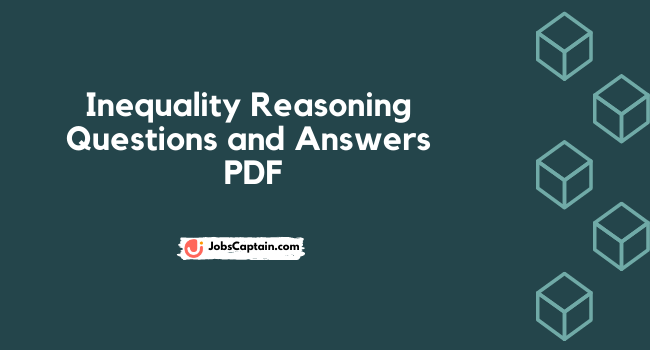# Inequality Reasoning Questions and Answers PDF [Inequalities Quiz]

By | April 14, 2021Inequality Reasoning Questions and Answers PDF includes all questions asked in previous year examinations. New Pattern Reasoning inequality questions notes gives shortcut tricks to remember difficult formulas.

Coded inequality reasoning questions PDF is useful in various competitive exams like IBPS, SBI, UPSC, Railway, RBI Asst., LIC Asst and RRB etc.

Inequalities Questions Pdf for IBPS, SBI PO/Clerk

50 Important Equality Inequality Questions PDF for IBPS PO Prelims

50+ Questions set of Inequalities Reasoning
Important Question with Answer Inequality for IBPS PO

In Inequalities Questions, statement containing different inequality symbols such as Greater than (>), Less than (<), Equals to (=), Greater than or Equals to (≥), and Less than or Equals to (≤).

You have to decode these symbols and answer whether the given relationship of the elements in a statement is true or not. To answer such questions you need to know the basic rules of the mathematical inequalities.

### Important Rules for Inequality Reasoning

• A relationship can be easily established between two elements if they have similar signs.
• A relationship cannot be easily established between two elements if they don’t have similar signs. In these cases, you have to put extra care-seeking either-or cases type conclusions.

### Types of Inequalities questions

(1)Direct Inequality:

Direct inequality is the symbols that are directly given in the statement.

(2) Coded Inequality:

Coded Inequality is the coded symbols provided in the statement and they will give their signification to the inequality symbol.

### Symbols used in Inequality

• a < b means a is lesser than b
• a ≤ b means a is less than or equal to b
• a = b means a is equal to b
• a ≥ b means a is greater than or equal to b
• a > b means a is greater than b
• a ≠ b means a isn’t equal to b

### Properties of Inequalities

 Property ≥ ≤ Addition If a ≥ b, then a + c ≥ b + c If a ≤ b, then a + c ≤ b + c Subtraction If a ≥ b, then a – c ≥ b – c If a ≤ b, then a – c ≤ b – c Multiplication If a ≥ b, then ac ≥ bc, where c>0 If a ≤ b, then ac ≤ bc, where c>0 Division If a ≥ b, then a/c ≥ b/c, where c>0 If a ≤ b, then a/c ≤ b/c, where c>0 Transitivity If a ≥ b and b ≥ c then, a ≥ c If a ≤ b and b ≤ c then, a ≤ c Inverse Additive If a ≥ b, then -a ≤ -b, if a>0, b>0 If a ≤ b, then -a ≥ -b, if a>0, b>0 Multiplicative If a ≥ b, then 1/a ≤ 1/b, if a>0, b>0 If a ≤ b, then 1/a ≥ 1/b, if a>0, b>0

Inequalities Questions for SBI Clerk, IBPS Clerk, RBI Asst., LIC Asst. and IBPS RRB Exams.

Moreover, All kinds of important Pdf notes and around 10,00,000 questions and complete General Knowledge Material can be found here. Subject wise Download Links for Handwritten Class Notes and Quizzes in Hindi and English.

Subject Wise Study Notes and Quizzes

 Computer Awareness Notes – Open General Hindi Notes – Open Reasoning Notes – Open English Grammar Notes – Open Maths Notes – Open Economics Notes – Open General Science Notes – Open Indian Polity Notes – Open History Notes – Open Today History in Hindi – Open Environment GK Notes – Open Banking GK in Hindi – Click Geography Notes – Open Computer Gk in Hindi – Open JEE and NEET Notes – Open Class 11 & 12 Science – Open English Grammar Quizzes – Play Now General Knowledge Quiz – Play Now

Disclaimer: All information’s / materials available on this website or the links provided on the site are for educational and study purposes only. We provide the links which is already available on the internet. The content is meant for individual and non-commercial uses only. We don’t want to violate any copyright law. These Pdf Notes provided for students who are financially troubled but deserving to learn. Thank you!

Category: Reasoning PDF